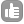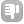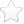1For spin ½ The echo trajectory M(t ) fits well to the function : M (t) =M0 * EXP (-t/T) * COS (pi * j * t) (t: spin-echo time). Can someone help me to find the equation that correspond to nuclei with spin 3/2 . asked Jul 26 '13 at 08:36ghNMR 11

Be the first one to answer this question!